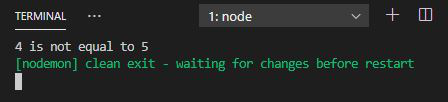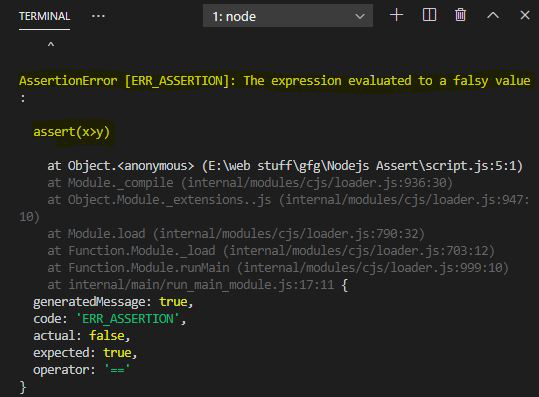Related Articles

# Node.js | Assert module

• Last Updated : 24 Jun, 2020

Assert module in Node.js provides a bunch of facilities that are useful for the assertion of the function. The assert module provides a set of assertion functions for verifying invariants. If the condition is true it will output nothing else an assertion error is given by the console.

Install the assert module using the following command:

`npm install assert`

Note: Installation is an optional step as it is inbuilt Node.js module.

Importing module:

`const assert = require("assert");`

Example 1:

 `console.clear()``const assert = require(``'assert'``);`` ` `let x = 4;``let y = 5;`` ` `try` `{`` ` `    ``// Checking condition``    ``assert(x == y);``}``catch` `{`` ` `    ``// Error output``    ``console.log(``        ```\${x} is not equal to \${y}`);``}`

Output:Example 2:

 `console.clear()``const assert = require(``'assert'``);`` ` `let x = 4;``let y = 5;`` ` `assert(x > y);`

Note: In this example, no try-catch is given so an assertion error of the kind given below will be the output.

Output:Note: Text Highlighted is the assertion error.

My Personal Notes arrow_drop_up